# A quick look into the Sktime for time-series forecasting (codes included)

Utpal Kumar   5 minute read

I used the sktime library to forecast the airline data using NaiveForecaster, KNeighborsRegressor, Statistical forecasters, and auto ARIMA model.

In this post, I will explore one of such Libraries that is rising real fast as a popular choice for Time series analysis in recent days - sktime. Sktime is a relatively new library in machine learning designed specifically for the time series. It is based on the scikit-learn library that I are familiar with but it has been tweaked for the time series. There are several approaches for machine learning in the Time series but the sktime takes a good bit from many different libraries and builds an efficient and compelling interface. So in my view, it could be a great starting point.

The goal is to try out several algorithms for forecasting time series using sktime.

## Install Sktime

Sktime has a good documentation for installation. The two approaches they suggest on their GitHub page are through pip and anaconda.

pip install sktime


I prefer the Anaconda way so I will use that but either way’s equally valid.

conda install -c conda-forge sktime


You may also need other libraries like matplotlib, seaborn,for running all the codes below.

You can simply install that by using the following command:

conda install -c conda-forge matplotlib seaborn
pip install pmdarima


## Data: Airline dataset

Let us first start with the data set that has become a standard for testing any subroutines in programming and data science. We will use the Box-Jenkins univariate airline data set, which shows the number of international airline passengers per month from 1949 - 1960. We use the exact dataset that has also been used by the Sktime documentation.

from sktime.datasets import load_airline
import matplotlib.pyplot as plt
y = load_airline() #y is pandas series object

fig, ax = plt.subplots(1, figsize=(10, 4))
ax.plot(y.values) #I convert that into numpy array
plt.savefig('airline-data-plot.png', dpi=300, bbox_inches='tight')
plt.close('all')


Now I perform the standard technique of splitting the data set into the training and test parts. The train part is used to build the model and the test part is used to “test” the result of training. Sktime provides a module to do that in an efficient way. This is based on the SKlearn module but has been a tweak to work specifically for time series.

from sktime.forecasting.model_selection import temporal_train_test_split
y_train, y_test = temporal_train_test_split(y, test_size=36)

fig, ax = plt.subplots(1,1, figsize=(10, 4))
y_train.plot(ax=ax, label='y_train')
y_test.plot(ax=ax, label='y_test')
plt.legend()
plt.savefig('train-test-airline-data-plot.png', dpi=300, bbox_inches='tight')
plt.close('all')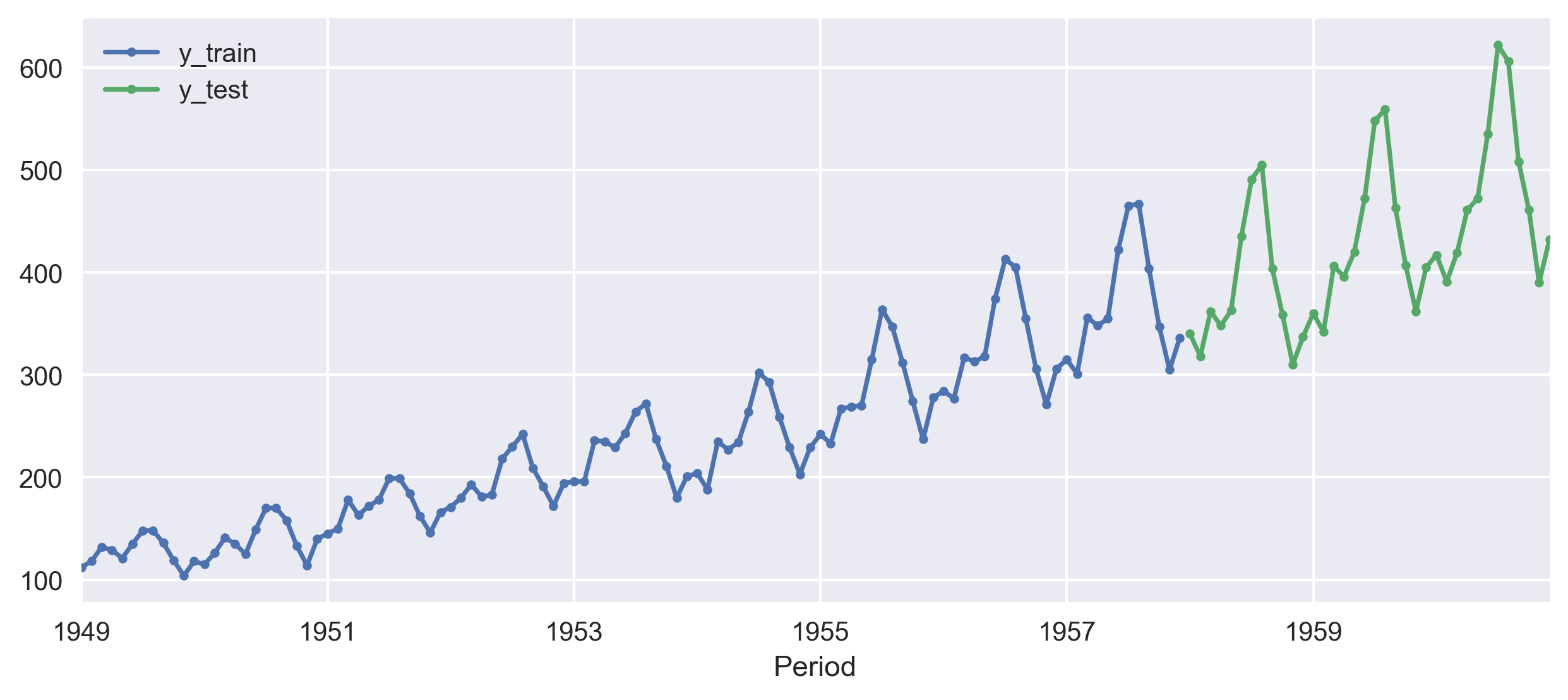Splitting airline dataset into test and train parts

Here, I will try to predict the last 3 years of data (hence the test size of 12*3 = 36), using the previous years as training data.

## Analysis

Now, I need to generate a forecasting horizon to use in the algorithm for forecasting. For this case, I’re interested in predicting from the first to the 36th step ahead because I used 36 data points as our test part.

import numpy as np
fh = np.arange(len(y_test)) + 1  # forecasting horizon


Similar to many other machine learning modules, in order to make forecasts, I need to first build a model, then fit it to the training data, and finally call predict to generate forecasts for the given forecasting horizon.

### NaiveForecaster

We will use NaiveForecaster to make forecasts using simple strategies.

from sktime.forecasting.naive import NaiveForecaster
from sktime.performance_metrics.forecasting import smape_loss

forecaster = NaiveForecaster(strategy="last", sp=12)
forecaster.fit(y_train)
y_pred = forecaster.predict(fh)
print(smape_loss(y_pred, y_test))

fig, ax = plt.subplots(1, 1, figsize=(10, 4))
y_train.plot(ax=ax, label='y_train', style='.-')
y_test.plot(ax=ax, label='y_test', style='.-')
y_pred.plot(ax=ax, label='y_predict', style='.-')
plt.legend()
plt.savefig('predict-airline-data-plot.png', dpi=300, bbox_inches='tight')
plt.close('all')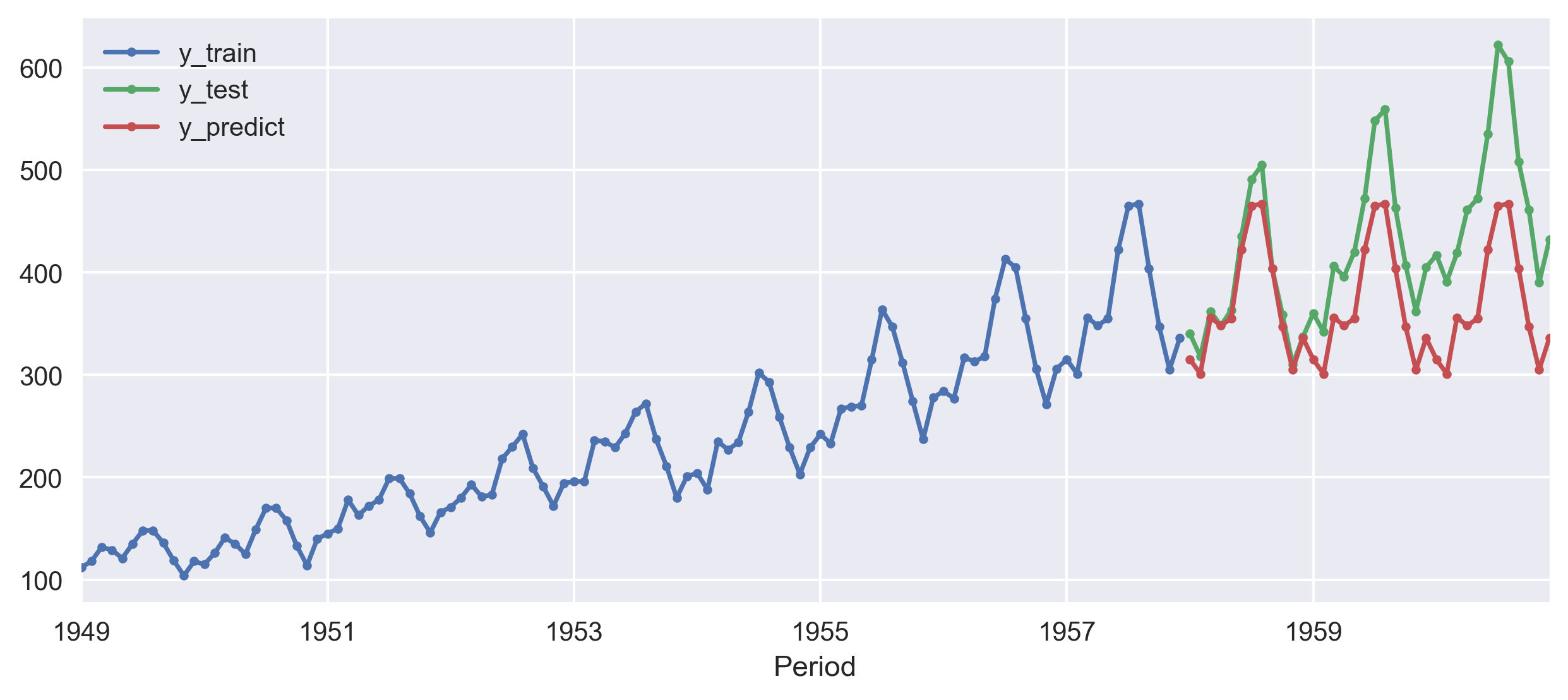Airline forecast using NaiveForecaster with strategy last and seasonal periodicity of 12.

In the above code, I chose the strategy for the forecast to be last, and seasonal periodicity of 12. Also, I used the sMAPE (symmetric mean absolute percentage error) to quantify the accuracy of our forecasts. A lower sMAPE means higher accuracy.

There are other popular performance evaluation metrics available for regression problems such as Mean Absolute Percentage Error (MAPE), Mean Absolute Scaled Error (MASE), Mean Directional Accuracy (MDA), etc, and I will investigate them in future posts. The other available strategies are drift and mean but since our data has an increasing trend so those may not be a good strategy.

In this case, I get the smape_loss to be 0.1454 for the last strategy but if I use mean then the prediction will go worse and I can see that reflected from the smape_loss value of 0.5908.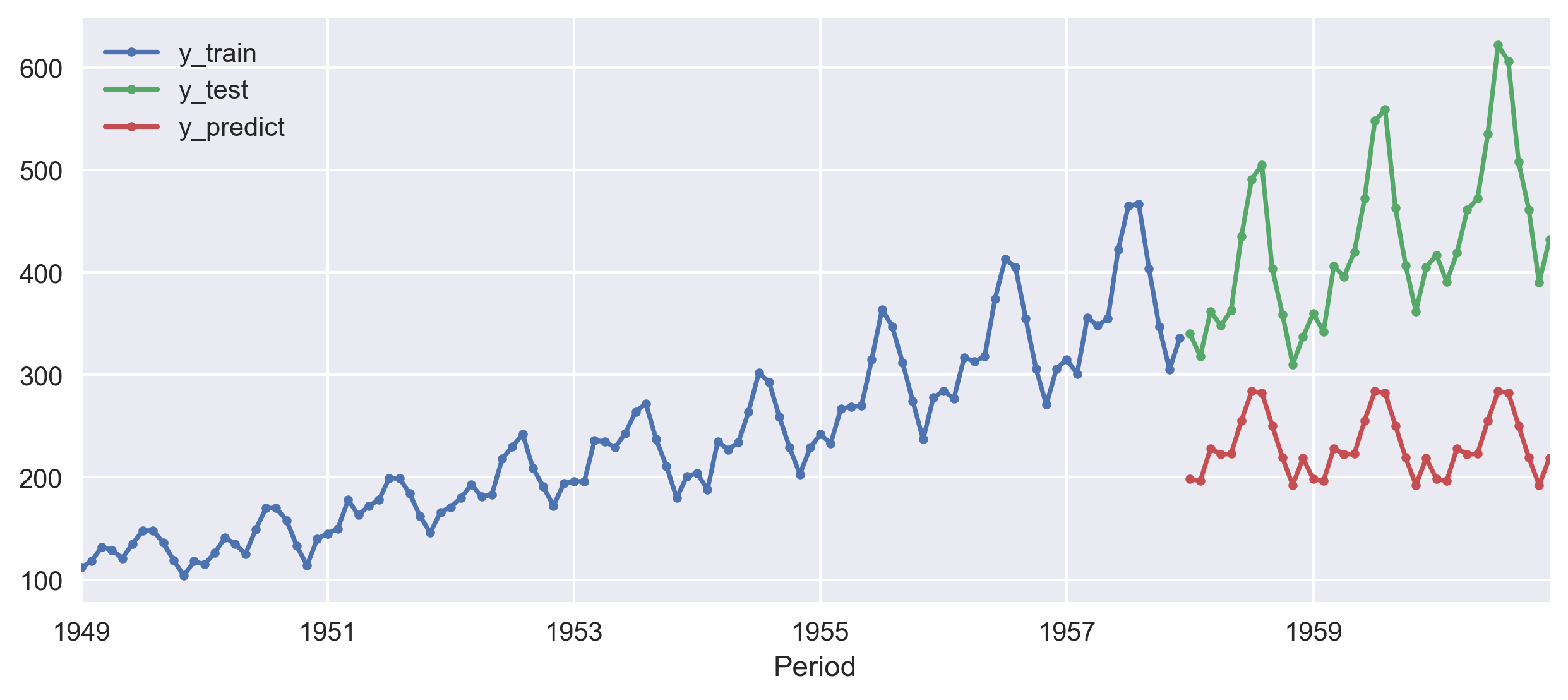Airline forecast using NaiveForecaster with strategy mean and seasonal periodicity of 12. The smape_loss is 0.5908

### KNeighborsRegressor

Now, let us use the regression based on k-nearest neighbors to forecast the time series. Here, I use the KNeighborsRegressor from the scikit-learn library. This predicts the target by local interpolation of the targets associated of the nearest neighbors in the training set.

from sktime.forecasting.compose import ReducedForecaster
from sklearn.neighbors import KNeighborsRegressor

regressor = KNeighborsRegressor(n_neighbors=1)
forecaster = ReducedForecaster(
regressor, scitype="regressor", window_length=15, strategy="recursive"
)
forecaster.fit(y_train)
y_pred = forecaster.predict(fh)
print(smape_loss(y_test, y_pred))

fig, ax = plt.subplots(1, 1, figsize=(10, 4))
y_train.plot(ax=ax, label='y_train', style='.-')
y_test.plot(ax=ax, label='y_test', style='.-')
y_pred.plot(ax=ax, label='y_predict', style='.-')
plt.legend()
plt.savefig('predict-KNeighborsRegressor-airline-data-plot.png',
dpi=300, bbox_inches='tight')
plt.close('all')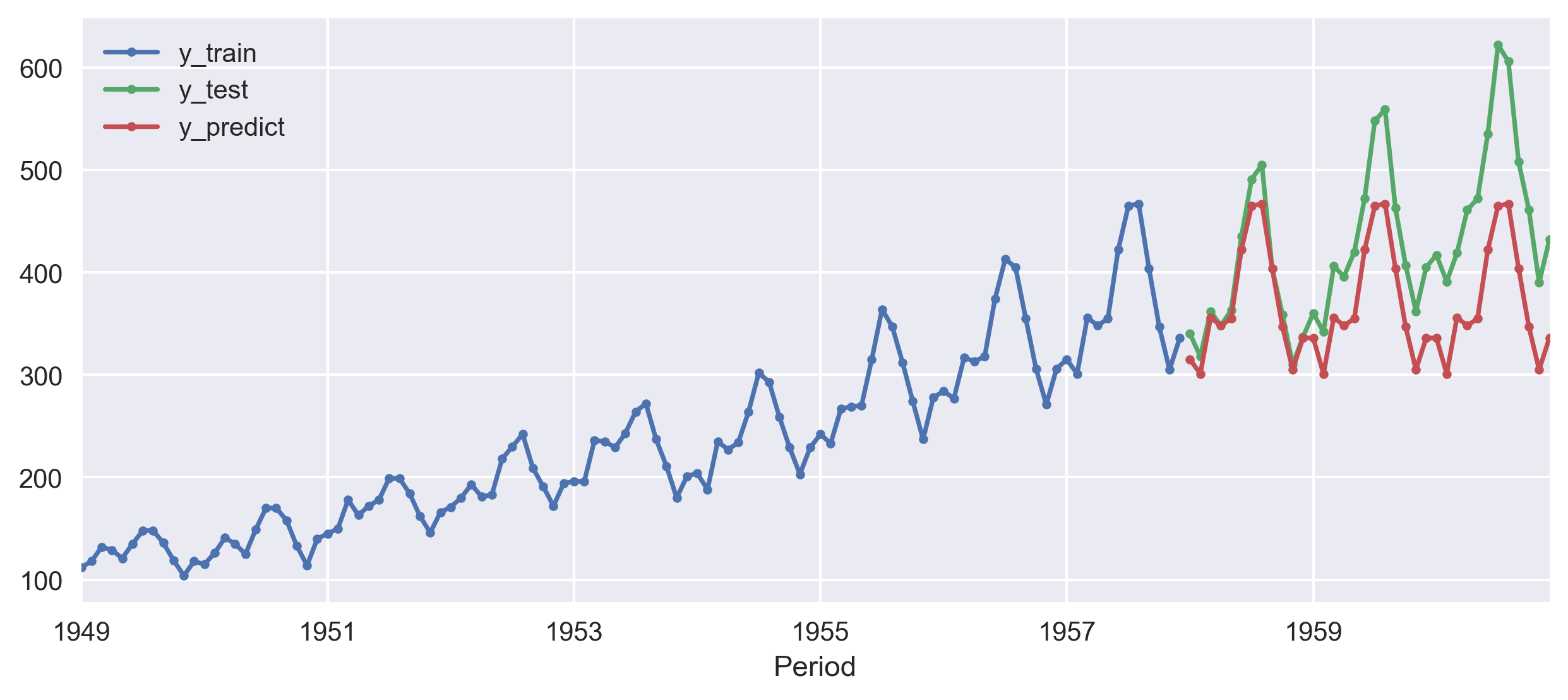Airline forecast using KNeighborsRegressor. The smape_loss in this case is 0.1418

The smape_loss, in this case, is 0.1418. We got a slight improvement from the NaiveForecaster but the difference is not substantial.

### Statistical forecasters

sktime also offers a number of statistical forecasting algorithms, based on implementations in statsmodels. We can then specify exponential smoothing with an additive trend component and multiplicative seasonality.

from sktime.forecasting.exp_smoothing import ExponentialSmoothing

forecaster.fit(y_train)
y_pred = forecaster.predict(fh)
print(smape_loss(y_test, y_pred))

fig, ax = plt.subplots(1, 1, figsize=(10, 4))
y_train.plot(ax=ax, label='y_train', style='.-')
y_test.plot(ax=ax, label='y_test', style='.-')
y_pred.plot(ax=ax, label='y_predict', style='.-')
plt.legend()
plt.savefig('predict-ExponentialSmoothing-airline-data-plot.png',
dpi=300, bbox_inches='tight')
plt.close('all')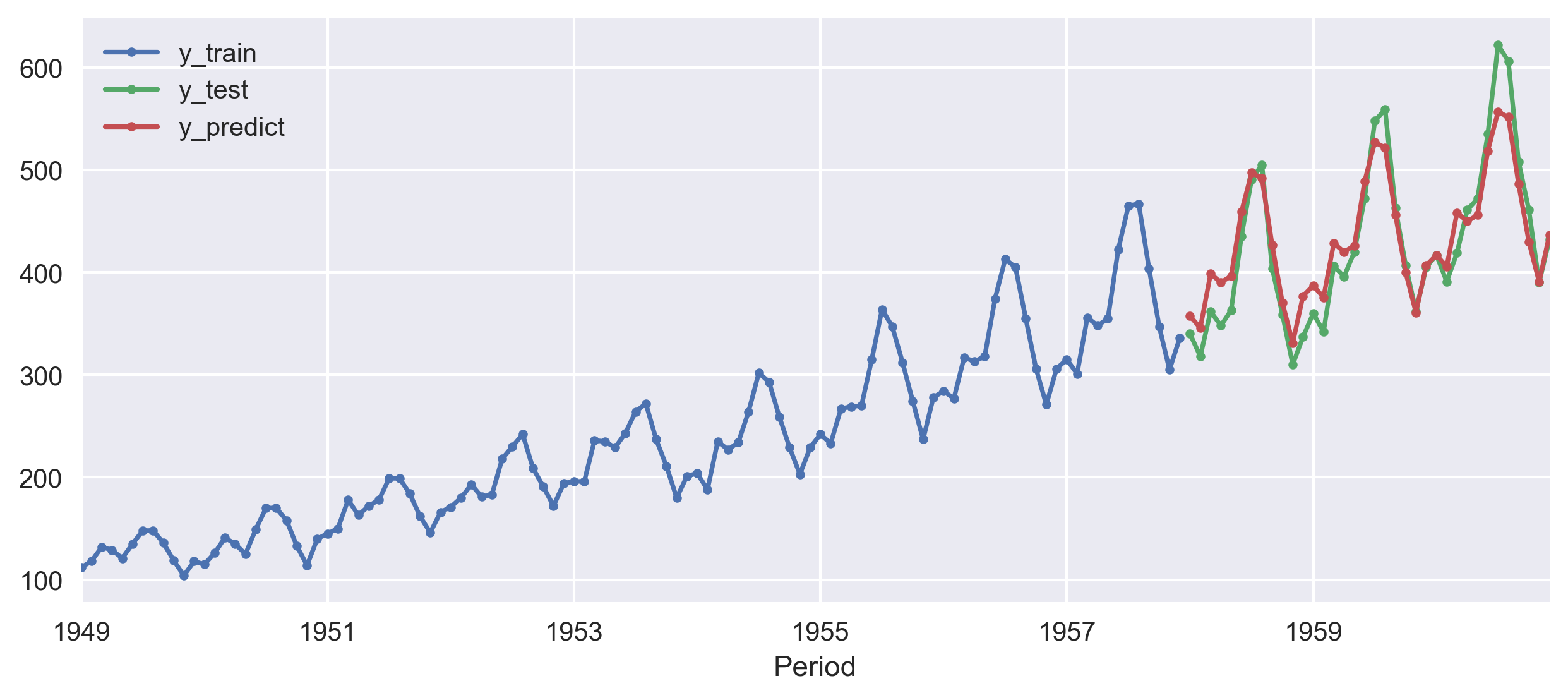Airline forecast using ExponentialSmoothing. The smape_loss in this case is 0.0502.

### Auto selected best ARIMA model

Sktime interfaces with pmdarima, a package for automatically selecting the best ARIMA model. This since searches over a number of possible model parametrizations, it is usually a bit slow.

from sktime.forecasting.arima import AutoARIMA

forecaster = AutoARIMA(sp=12, suppress_warnings=True)
forecaster.fit(y_train)
y_pred = forecaster.predict(fh)
print(smape_loss(y_test, y_pred))

fig, ax = plt.subplots(1, 1, figsize=(10, 4))
y_train.plot(ax=ax, label='y_train', style='.-')
y_test.plot(ax=ax, label='y_test', style='.-')
y_pred.plot(ax=ax, label='y_predict', style='.-')
plt.legend()
plt.savefig('predict-AutoARIMA-airline-data-plot.png',
dpi=300, bbox_inches='tight')
plt.close('all')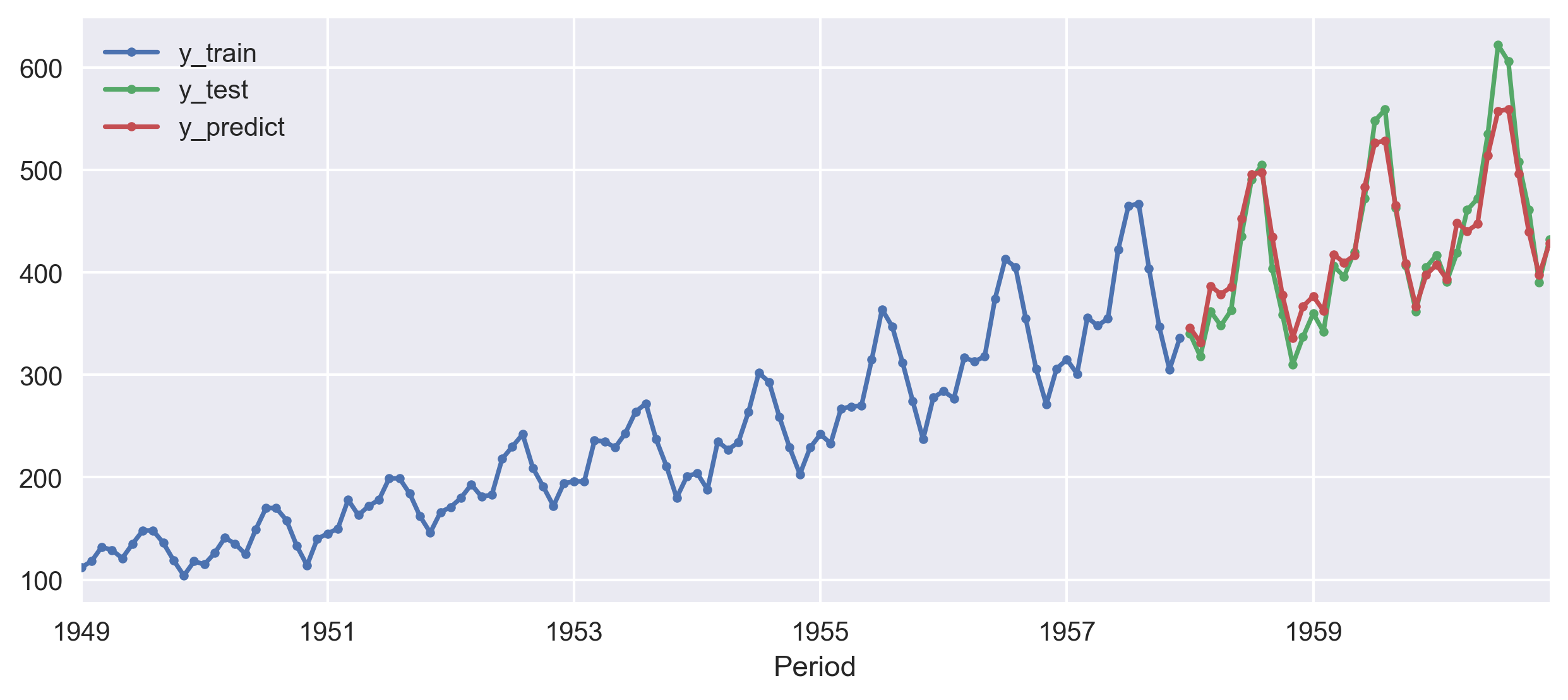Airline forecast using AutoARIMA. The smape_loss in this case is 0.0411.

## Conclusions

Sktime is a promising library for machine learning applications for time series and has advantages over using lower-level libraries such as Sklearn. Also, as it interfaces with several other mature machine learning libraries in Python, it can be used to efficiently employ algorithms from sklearn or pmdarima directly for the time series analysis.

This tutorial is simple in nature and the future posts will cover the advanced applications of sktime as I get more into it. Sktime also has a powerful library for univariate time series classification analysis. We will cover that in future posts.

Also, I will look into the other popular libraries for time series analysis such as tslearn (for classification/clustering), pyts (for classification), statmodels (forecasting and time series analysis), gluon-ts (forecasting, anomaly detection), tsfresh (feature extraction), etc.

1. Markus Löning, Anthony Bagnall, Sajaysurya Ganesh, Viktor Kazakov, Jason Lines, Franz Király (2019): “sktime: A Unified Interface for Machine Learning with Time Series”
2. Forecasting with sktime